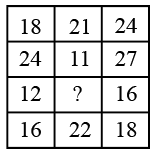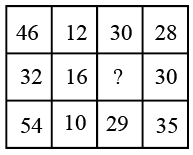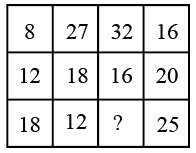# Delhi SI CPO Nov 25 Shift-1

Instructions

For the following questions answer them individually

Question 1

# In a certain coding system, ‘Read This book’ is coded as ‘689', ‘This Book Is useful’ is coded as ‘9675’, and ‘Useful Book is good' is coded as ‘5479'. What is the code for ‘This Book Is good’ in this system ?Question 2

# Identify the number which when added to itself 14 times gives 195.Question 3

# Study the given pattern carefully and select the number that can replace the question mark (?) in it.Question 4

# Which letter-cluster will replace the question mark (?) in the following series ?SAG, KSY, CKQ, ?Question 5

# In a family of five members,P is Q’s sister and R is Q’s mother. S is R’s father and T is S’s mother. How is P related to S ?Question 6

# Study the given pattern carefully and select the number that can replace the question mark (?) in it.Question 7

# $$X$$ is $$7^{th}$$ from the top while $$Y$$ is $$16^{th}$$ from the bottom in the class ranking. If they interchange their positions, then $$X$$ becomes $$21^{st}$$ from the top. What will be the position of $$Y$$ from the bottom?Question 8

# Which two numbers and two signs can be interchanged so as to balance the given equation?$$16\times4+12\div4-15=59$$Question 9

# Study the given pattern carefully and select the number that can replace the question mark (?) in it.Question 10

# In a certain coding system, if OXBRIDGE is written as BDEGIORX, how will MOUTHFUL be written in the same coding system ?OR